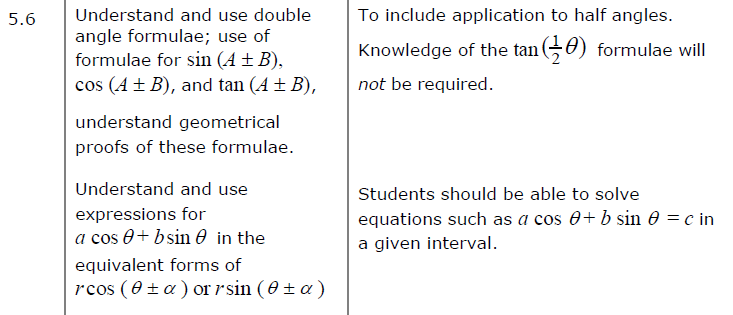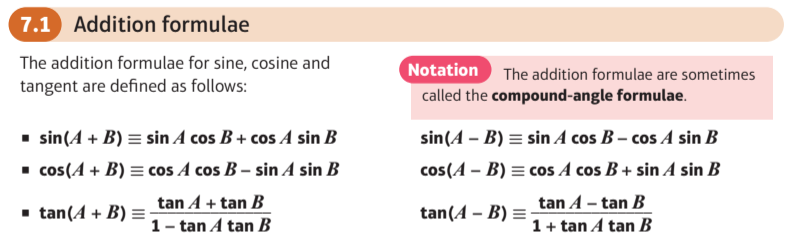Instant Maths Help:

# Edexcel A Level - Addition Formulae

## Trig Addition Formulae & Double Angle Formulae##### Prove addition formulae using geometric construction##### Visualise sin(2x) Double Angle Formulae
The double angle formulae are useful in solving trigonometric formulae. This display allows you to view formulae relating to $\color{green}{\sin (a \, x)}$ with $\color{green}{a}$ an adjustable constant.

Initially, $\color{green}{a}$ is set to 0 so you can visualise how $\color{red}{\sin (c \, x) \cos (c \, x)}$ arises as the product of $\color{grey}{\sin (c \, x)}$ and $\color{grey}{\cos (c \, x)}$.

Then, by adjusting $\color{green}{a}$, $\color{red}{b}$ and $\color{red}{c}$ to make the green and red curves coincide, you can view the double angle formulae, not only for $\color{green}{\sin (2 \, x)}$, but for other values of $\color{green}{a}$ too.
##### Visualise cos(2x) Double Angle Formulae
The double angle formulae are useful in solving trigonometric formulae. This display allows you to view formulae relating to $\color{green}{\cos (a \, x)}$ with $\color{green}{a}$ an adjustable constant.

By adjusting $\color{green}{a}$, $\color{red}{b}$, $\color{red}{c}$ and $\color{red}{d}$ to make the green and red curves coincide, you can view the double angle formulae, for $\color{green}{\cos (2 \, x)}$ in its different forms.
##### Visualise sin(x ${ \pm }$ a) Addition Formulae
This display allows you to visualise the addition formulae:
$\color{purple}{ \sin (x + a) = \sin(x) \cos(a) + \cos(x) \sin(a) }$
$\color{purple}{ \sin (x - a) = \sin(x) \cos(a) - \cos(x) \sin(a) }$
##### Visualise cos(x ${ \pm }$ a) Addition Formulae
This display allows you to visualise the addition formulae:
$\color{purple}{ \cos (x + a) = \cos(x) \cos(a) - \sin(x) \sin(a) }$
$\color{purple}{ \cos (x - a) = \cos(x) \cos(a) + \sin(x) \sin(a) }$
##### Visualise a sin x ${ \pm }$ b cos x to sin
This display allows you to visualise the transformations:
$\color{purple}{ a \, \sin (x) + b \, \cos (x) = R \, \sin (x + \alpha) }$
$\color{purple}{ a \, \sin (x) - b \, \cos (x) = R \, \sin (x - \alpha) }$

After adjusting $\color{red}{a}$ and $\color{blue}{b}$, you can adjust $\color{green}{r}$ and $\color{green}{\alpha}$ to their derived values to see the two curves coincide.

For a better view, you should use the navigation buttons in the lower right corner to zoom in and out.
##### Visualise a cos x ${ \pm }$ b sin x to cos
This display allows you to visualise the transformations:
$\color{purple}{ a \, \cos (x) + b \, \sin (x) = R \, \cos (x - \alpha) }$
$\color{purple}{ a \, \cos (x) - b \, \sin (x) = R \, \cos (x + \alpha) }$

After adjusting $\color{red}{a}$ and $\color{blue}{b}$, you can adjust $\color{green}{r}$ and $\color{green}{\alpha}$ to their derived values to see the two curves coincide.

For a better view, you should use the navigation buttons in the lower right corner to zoom in and out.
##### sin(x + a) + sin(x - a)
The display shows $$\color{blue}{y = \sin x}$$ in blue and $$\color{red}{y = \sin \left( {x + a} \right) + \sin \left( {x - a} \right)}$$